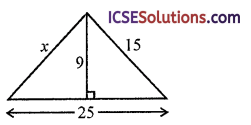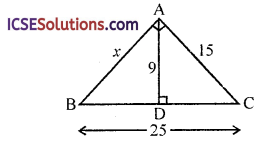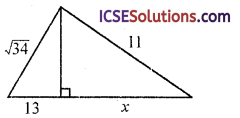Access to comprehensive ICSE Class 9 Maths Solutions S Chand Chapter 10 Pythagoras Theorem Chapter Test encourages independent learning.

## S Chand Class 9 ICSE Maths Solutions Chapter 10 Pythagoras Theorem Chapter Test

We know that in a right angled triangle (hypotenuse)² = sum of squares on other two sides.

Question 1.
Find the value of x.Solution:
In the figure, the triangle is right angled
∴ x² = 62 + 82 = 36 + 64 = 100 = (10)²
∴ x = 10

Question 2.Solution:
In the figure, the triangle is a right angled
∴ 7² = x² + 2² ⇒ 49 = x² + 4
⇒ x² = 49 – 4 = 45 = 9 x 5
x = $$\sqrt{9 \times 5}$$ = 3 $$\sqrt{5}$$Question 3.Solution:
In the figure, two triangles are right angledIn right ∆ABC
⇒ 152 = 92 + DC² ⇒ 225 = 81 + DC²
⇒ DC² = 225 – 81 = 144 = (12)²
∴ DC = 12
But BC = 25
∴ BD = BC – DC = 25 – 12 = 13
Now in right ∆ABD
x² = 92 + 132 = 81 + 169 = 250
∴ x = $$\sqrt{250}=\sqrt{25 \times 10}=5 \sqrt{10}$$

Question 4.Solution:
In the figure, two triangles are right angledIn ∆ABD
($$\sqrt{34}$$)² = AD² + 132 ⇒ 34 = AD² + 169
Tell if the measures can be the side lengths of a triangle. If so classify the triangle as acute, obtuse, or right.
Note: We know, the sum of any two sides of a triangle is greater than its third side and
(Hypotenuse) = Sum of squares of other two sides.

Question 5.
10, 22, 15
Solution:
Sides are 10, 22, 15
Here 10 + 15 > 22
∴ It is a triangle.
Now (longest side)² = (22)² = 484
Sum of square of other two sides = 10² + 15²
= 100 + 225 = 325
∴ 484 > 325
∴ It is an acute triangle.Question 6.
8, 13, 23
Solution:
8, 13, 23
∵ 8 + 13 is not greater than 23
∴ These are not sides of a triangle

Question 7.
9, 14, 17
Solution:
9, 14, 17
∵9 + 14 > 17
∴ It is a triangle
Now, (17)² = 289
and 9² + 142 = 81 + 196 = 277
∵ 289 > 277
∴ It is an obtuse angled triangle.

Question 8.
1$$\frac { 1 }{ 2 }$$, 2, 2$$\frac { 1 }{ 2 }$$
Solution:
1$$\frac { 1 }{ 2 }$$, 2, 2$$\frac { 1 }{ 2 }$$
⇒ $$\frac { 3 }{ 2 }$$, 2, $$\frac { 5 }{ 2 }$$
⇒ 1.5, 2, 2.5
∵ 2 + 1.5 > 2.5
It is a triangle.
Now, (2.5)² = 6.25
and 2² + 1.52 = 4 + 2.25 = 6.25
∵ 6.25 = 6.25
It is a right angled triangle.

Question 9.
Two trees are standing on flat ground. The height of the smaller tree is 7 m. The distance between the top of the smaller tree and the base of the taller tree is 15 m. The distance between the top of the taller tree and the base of the smaller tree is 20 m. Calculate(i) the horizontal distance between the two trees.
(ii) the height of the taller tree.
Solution:
There are two trees on a flat ground
Height of smaller tree = 7 m
Let AB and CD are two trees, AB = 7 m
Distance between their tops and foot of other are 15 m and 20 m
Let h be the height of bigger tree, DB is the distance between their feet = x mAD² = AB² + DB² (Pythagoras theorem)
⇒ 15² = 72 + x²
⇒ 225 = 49 + x²
⇒ x² = 225 – 49 = 176
∴ x = $$\sqrt{176}=\sqrt{16 \times 11}=4 \sqrt{11}$$

(ii) and in right ∆BCD
BC² = CD² + DB²
⇒ 20² = h² + 176
⇒ 400 = h² + 176
⇒ h² = 499 – 176 = 224
⇒ h = $$\sqrt{224}=\sqrt{16 \times 14}$$
= 4 $$\sqrt{14}$$ mQuestion 10.
A girl walks 200 m towards East and then 150 m towards North. The distance of the girl from the starting point is
(a) 350 m
(b) 250 m
(c) 300 m
(d) 225 m
Solution:
A girl walks 200 m towards East then 150 m towards North
i.e., OA = 200 m and AB = 150 m∴ OB² = OA² + AB²
= (200)² + (150)² = 40000 + 22500
= 62500 = (250)²
OB = 250 m